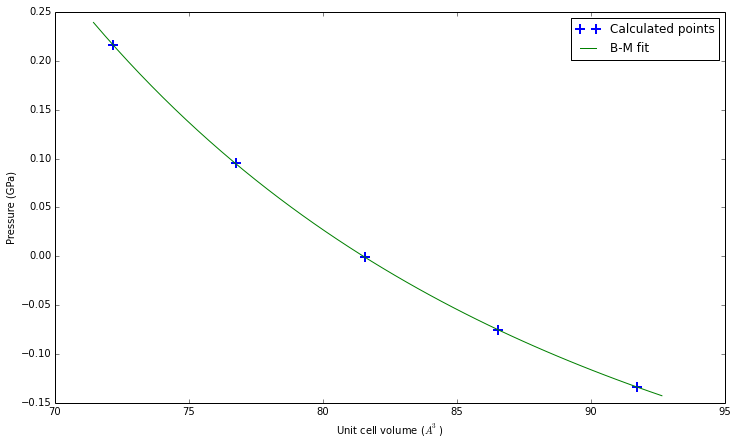# Calculating elastic constants¶

Read previous tutorials to understand all material presented here.

The initial setup is identical to the one used in "Remote calculation". Scroll down below first cell for the material of the tutorial.

In :
# Import the basic libraries

# ASE system
import ase
from ase import Atom, Atoms
from ase import io
from ase.lattice.spacegroup import crystal

# Spacegroup/symmetry library
from pyspglib import spglib

# iPython utility function
from IPython.core.display import Image

# Import the qe-util package
from qeutil import RemoteQE

# Access info
import host


## Elastic library¶

Calculation of elastic constans requires inclusion of additional library: elastic. This library extends standard crystal object, and provides new features related to elastic properties of the crystal. This library must be first installed in the system. See the Install document for the information how to do it. Here we just import the library. It must be imported before the creation of the first crystal.

## Calculator definition¶

Since the calculation of elastic constants requires deformation of the crystal the calculator must not try to use symmetry for the calculations. Otherwise each calculation will be done with different internal setup and the results will be inconsistent. Thus we pass the use_symmetry=False parameter to the calculator constructor. The calculation of elastic constants requires a large number of individual calculations to be performed, thus only RemoteQE calculator is suitable for this type of calculations.

In :
# Import additional library for elastic constants calculations
import elastic

In :
# Stup the SiC crystal
# Create a cubic crystal with a spacegroup F-43m (216)
a=4.3366
cryst = crystal(['Si', 'C'],
[(0, 0, 0), (0.25, 0.25, 0.25)],
spacegroup=216,
cellpar=[a, a, a, 90, 90, 90])

In :
qe=RemoteQE(label='SiC-elast',
kpts=[2,2,2],
xc='pz',         # Exchange functional type in the name of the pseudopotentials
pp_type='vbc',   # Variant of the pseudopotential
pp_format='UPF', # Format of the pseudopotential files
ecutwfc=70,
pseudo_dir='../pspot',
use_symmetry=False,
procs=8)         # Use 8 cores for the calculation

print "Local working directory:", qe.directory

Local working directory: calc/SiC-elast.NTcCnS

In :
# Assign the calculator to our system
cryst.set_calculator(qe)

In :
# Run the calculation to get stress tensor (in Voigt notation, GPa) and pressure (in GPa)
print "Stress tensor     (GPa):", cryst.get_stress()/ase.units.GPa
print "External pressure (GPa):", cryst.get_pressure()/ase.units.GPa

Stress tensor     (GPa): [ 0.149  0.149  0.149 -0.    -0.    -0.   ]
External pressure (GPa): -0.149


## Equation of state¶

The elastic library provides an equation of state calculation procedure, similar to the one we have used in the first tutorial. It automatically runs a number of single point calculations for given range of volumes (from lo to hi - the values are scaling factors). The default range [0.98,1.02] is equivalent to +/-2% compression/expansion. The data is collected and the Birch-Murnaghan equation of state:

$$P(V)=\frac{B_0}{B'_0} \left[\left(\frac{V_0}{V}\right)^{B'_0}-1\right]$$

is fitted to the data points.

In :
fit=cryst.get_BM_EOS(lo=0.96,    # lower bound of the volumes range
hi=1.04,    # higher bound of the volumes range
n=5)        # number of volume points used in the fit

print "\nA0=%.4f A  ;  B0=%.1f GPa  ;  B0'=%.2f " % (fit**(1.0/3), fit/ase.units.GPa, fit)

Launching: 1 2 3 4 5
Done: 1 2 3 4 5

A0=4.3358 A  ;  B0=224.8 GPa  ;  B0'=3.74


## Quality test¶

We can easily check the quality of the fit by plotting the fitted function together with the calculation data. The calculated data points are saved into the cryst.pv attribute of the crystal object. The array contains following data: volume, pressure, lattice constants: A,B,C.

In :
# Get the data from the crystal object
pv=cryst.pv.T

# Definition of the B-M EOS function
func = lambda v, v0, b0, b0p: (b0/b0p)*(pow(v/v0,-b0p) - 1)

figsize(12,7)
plot(pv,pv,'+',label='Calculated points',markersize=10,markeredgewidth=2)

v=linspace(min(pv)*0.99,max(pv)*1.01, 50)
plot(v, func(v,fit,fit,fit),label="B-M fit");
xlabel('Unit cell volume ($A^3$)')
ylabel('Pressure (GPa)')
legend();## Elastic constants¶

Elastic module provides mainly one central function: the procedure for calculation of elastic constants of a crystal. Since the calculation of elastic constants involves many individual calculations (from 10 to even hundreds) it is beneficial to have some control over the execution of individual calculations. Thus the get_elastic_tensor provides two modes of operation.

• Fully automatic (default) - does not provide any access to the component calculations. In case of any problems with the execution of individual jobs we have no chance to correct the situation. This may be very wastefull since the only option is to repeat the whole calculation. But the usage is extremely simple - just call cryst.get_elastic_tensor() to get the elastic tensor and Birch coefficients for the given structure.

• Staged mode - recommended, particularly for large systems with many individual jobs. This mode provides a three-step procedure:

1. Run cryst.get_elastic_tensor(mode='deformations') to get a list of deformed crystals to calculate separately.
2. Run elastic.ParCalculate(system_list,calculator) to calculate the stresses of individual structures
3. Pass the list of systems to the cryst.get_elastic_tensor(mode='restart',systems=system_list) to get the elastic tensor.

We will run the calculation in the staged mode which is safer and gives us a chance to intervene if something goes wrong.

In :
deformations=cryst.get_elastic_tensor(mode='deformations')

In :
elastic.ParCalculate(deformations,qe);

Launching: 1 2 3 4 5 6 7 8 9 10
Done: 1 2 3 4 5 6 7 8 9 10

In :
Cij,Bij=cryst.get_elastic_tensor(mode='restart',systems=deformations)


Finally we got the elastic tensor of the crystal. Depending on the symmetry of the crystal the number of constants changes. The general ordering of $C_{ij}$ components is (except for triclinic symmetry and taking into account customary names of constants - e.g. $C_{16} \rightarrow C_{14}$:

$$C_{11}, C_{22}, C_{33}, C_{12}, C_{13}, C_{23}, C_{44}, C_{55}, C_{66}, C_{16}, C_{26}, C_{36}, C_{45}$$

To get the ordering for your crystal drop from the above list the positions irrelevant for its symmetry. Thus for our cubic crystal the order of constants will be: $C_{11}, C_{12}, C_{44}$.

In :
print "Elastic tensor (GPa) [C_11, C_12, C_44]:", Cij/ase.units.GPa

Elastic tensor (GPa) [C_11, C_12, C_44]: [ 392.951       145.439       274.90982353]

In :